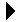Function Access# Function AccessRules for all pointers Download Integer Stack Package Specification```--
-- One of the classic examples of the use of procedural parameters is
-- a generalized sort.  Many languages support procedural parameters, excepting
-- Java, where such things are accomplished using interfaces.  In Ada,
-- procedural parameters are created by creating a function (or procedure)
-- access type.
--
-- This file implements a quicksort to sort a list of (X,Y) points.  It uses
-- a comparison function sent as a parameter to compare the points.  It then
-- sorts them in various ways with several such functions.
--
with Gnat.Io; use Gnat.Io;
procedure DoSort is
-- We're going to work with an array of x, y points.
type Point is record
X, Y: Integer;
end record;

-- This type describes a sort comparison function.
type Comparator is
access function (A, B: Point) return Integer;

-- Arrays of integers which can be sorted.
type Point_Arr is array (Positive range <>) of Point;

-- Print the array.
procedure Print(Arr: Point_Arr) is
begin
Put("[");
for I in Arr'Range loop
Put("(");
Put(Arr(I).X);
Put(",");
Put(Arr(I).Y);
Put(")");
end loop;
Put_Line("]");
end Print;

-- This is the sort function itself.  Because it's a nice use of
-- slices, it's a recursive quicksort.  This will sort an array of
-- integers in any order definable by a comparison function which
-- you send.
procedure Sort(Arr: in out Point_Arr; Comp: Comparator) is
-- This is the partition function for quick sort.
procedure Split(Pivot: out Integer; Arr: in out Point_Arr) is
-- A simple swap procedure is useful.
procedure Swap(A, B: in out Point) is
T: Point;
begin
T := A;
A := B;
B := T;
end;

-- These are scanners for the left and right ends of the
-- split algorithm.
Left: Integer := Arr'First + 1;
Right: Integer := Arr'Last;
begin
-- Put("In:  "); Print(Arr);

loop
-- Move the left pointer to the first item out of place on the
-- left side.
while Left < Arr'Last and then
Comp(Arr(Left), Arr(Arr'First)) <= 0 loop
Left := Left + 1;
end loop;

-- Move the right pointer to the first item out of place on the
-- left side.
while Right > Arr'First and then
Comp(Arr(Right), Arr(Arr'First)) > 0 loop
Right := Right - 1;
end loop;

-- If they pass, we are done.
exit when Left >= Right;

-- Swap, then go 'round again.
Swap(Arr(Left), Arr(Right));

end loop;

-- Put the pivot in place.
Swap(Arr(Right), Arr(Arr'First));
Pivot := Right;

end Split;

-- Location of the pivot point.
Pivot: Integer;
begin

-- See if this is large enough to need sorting.
if Arr'length > 1 then
Split(Pivot, Arr);

Sort(Arr(Arr'First..Pivot-1), Comp);
Sort(Arr(Pivot+1..Arr'Last), Comp);
end if;

end Sort;

-- Here are some comparisons which we can use with the sort.

-- Sort by X first, then Y for points with matching X.
function ByX(A, B: Point) return Integer is
Primary: Integer; -- Result of primary comparison.
begin
Primary := A.X - B.X;
if Primary /= 0 then
return Primary;
else
return A.Y - B.Y;
end if;
end;

-- Sort by Y first, then by X.
function ByY(A, B: Point) return Integer is
Primary: Integer; -- Result of primary comparison.
begin
Primary := A.Y - B.Y;
if Primary /= 0 then
return Primary;
else
return A.X - B.X;
end if;
end;

-- Distance from the origen.  (I probably don't really need to take the
-- the square root just to get the comparison.)
function Dist(A, B: Point) return Integer is
begin
-- Compute each distance using results from some old Greek guy, whose
-- stuff still works somehow even though we've invented computers.
-- Amazing!
Adist := Sqrt(Float(A.X*A.X + A.Y*A.Y));
Bdist := Sqrt(Float(B.X*B.X + B.Y*B.Y));

-- Compute and return the result.
if Adist < Bdist then
return -1;
elsif Adist > Bdist then
return 1;
else
return 0;
end if;
end;

-- This orders the points by position in a rotational sweep from the
-- positive X axis counter-clockwise.
function Sweep(A, B: Point) return Integer is
begin
-- Compute the angles from 0.0 using arc tangent.
Arad := Arctan(X => Float(A.X), Y => Float(A.Y));
Brad := Arctan(X => Float(B.X), Y => Float(B.Y));

-- Compute and return the result.
return -1;
return 1;
else
return 0;
end if;
end;

Pts: Point_Arr :=
((5, -8), (-4, 19), (1, 10), (10, 2), (-4, -4), (-4, 2), (3, 2), (10, 1));
begin
Sort(Pts, ByX'Access);
Put("Sort by X: ");
Print(Pts);
New_Line;

Sort(Pts, ByY'Access);
Put("Sort by Y: ");
Print(Pts);
New_Line;

Sort(Pts, Dist'Access);
Put("Sort by Dist: ");
Print(Pts);
New_Line;

Sort(Pts, Sweep'Access);
Put("Sort by Angle: ");
Print(Pts);
New_Line;
end DoSort;
```Rules for all pointers Integer Stack Package Specification